Скачать презентацию Algorithmic Game Theory Polynomial Time Algorithms and Internet

5f540f77b0d83e352d588dd583a9107d.ppt

• Количество слайдов: 58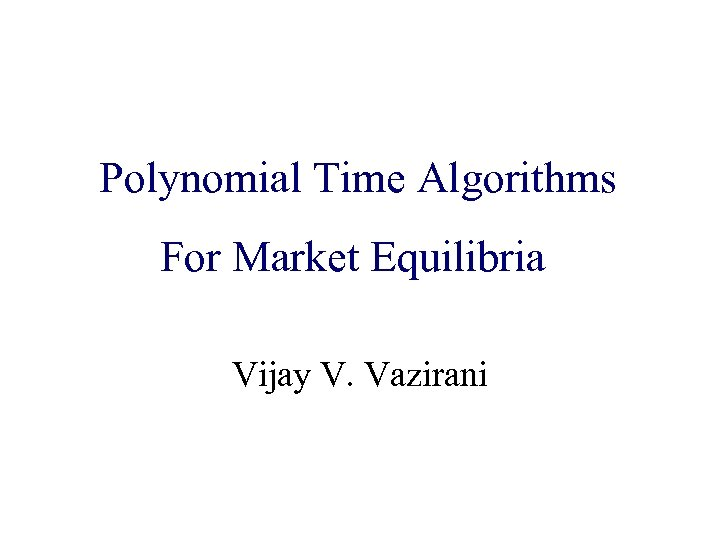Algorithmic Game Theory Polynomial Time Algorithms and Internet Computing For Market Equilibria Vijay V. Vazirani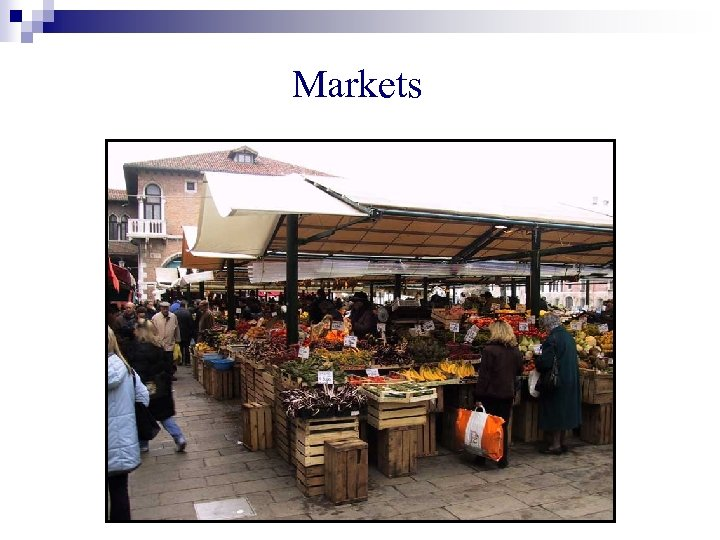MarketsStock Markets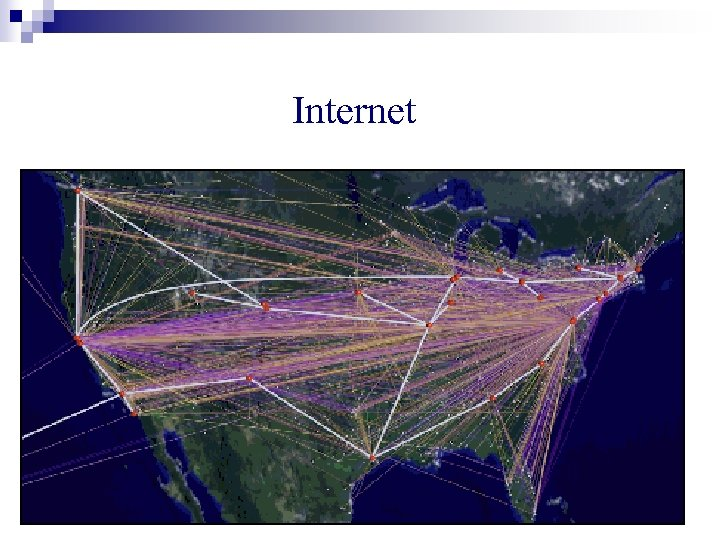Internet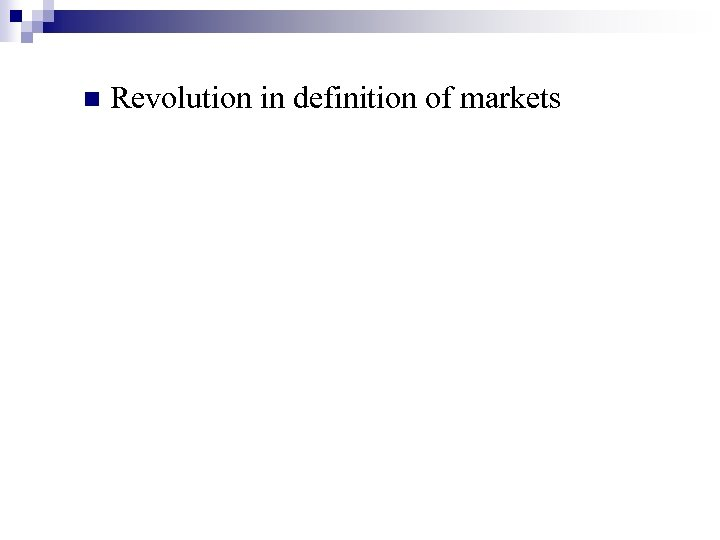n Revolution in definition of markets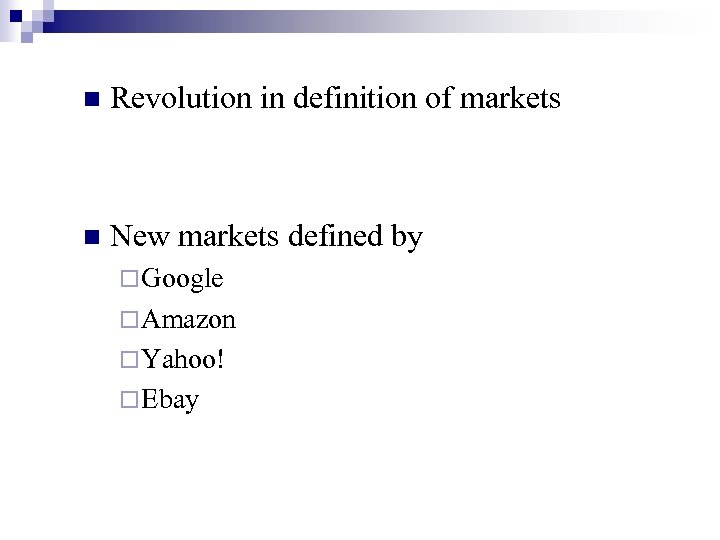n Revolution in definition of markets n New markets defined by ¨ Google ¨ Amazon ¨ Yahoo! ¨ Ebay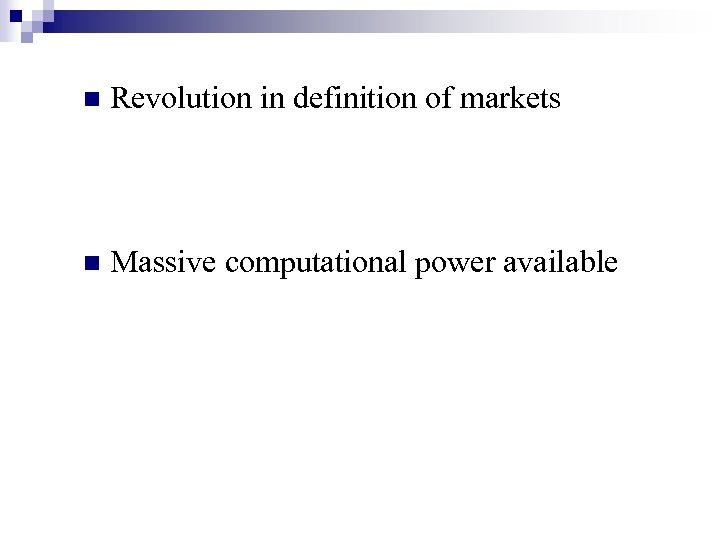n Revolution in definition of markets n Massive computational power available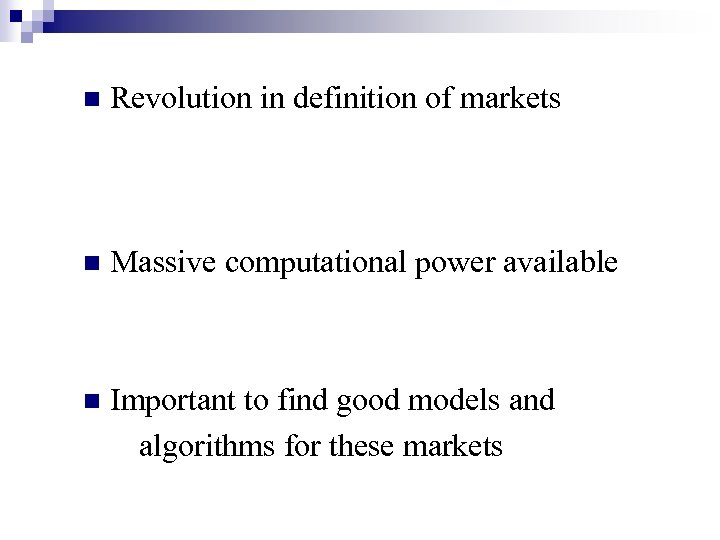n Revolution in definition of markets n Massive computational power available n Important to find good models and algorithms for these markets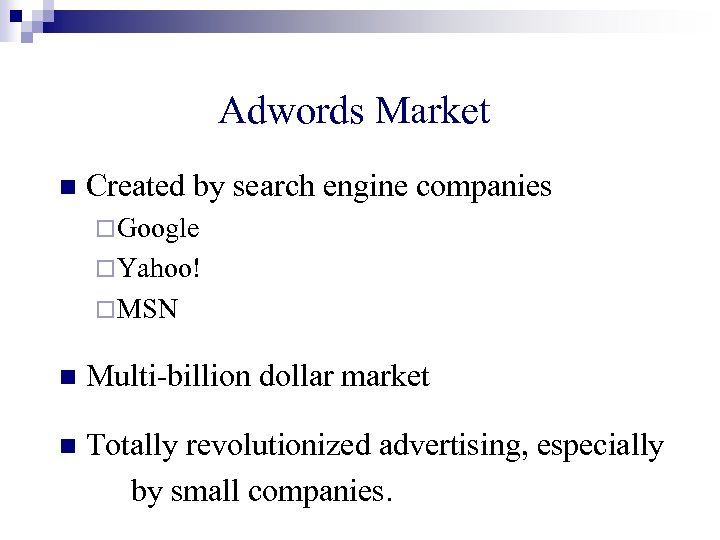Adwords Market n Created by search engine companies ¨ Google ¨ Yahoo! ¨ MSN n Multi-billion dollar market n Totally revolutionized advertising, especially by small companies.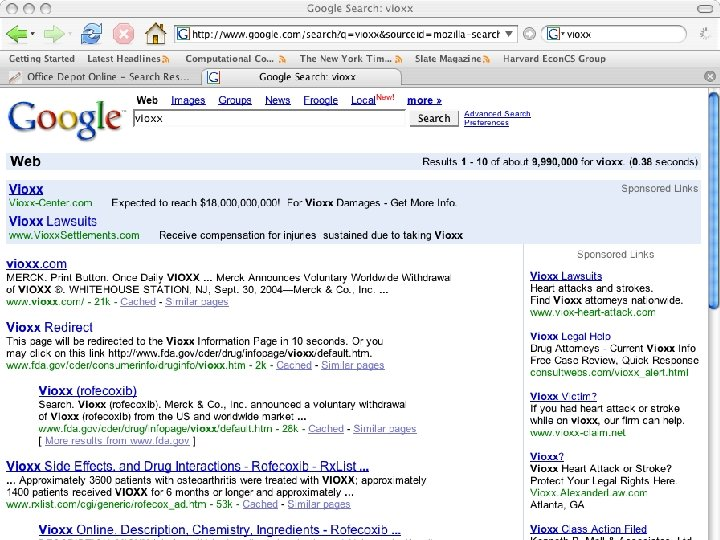How will this market evolve? ?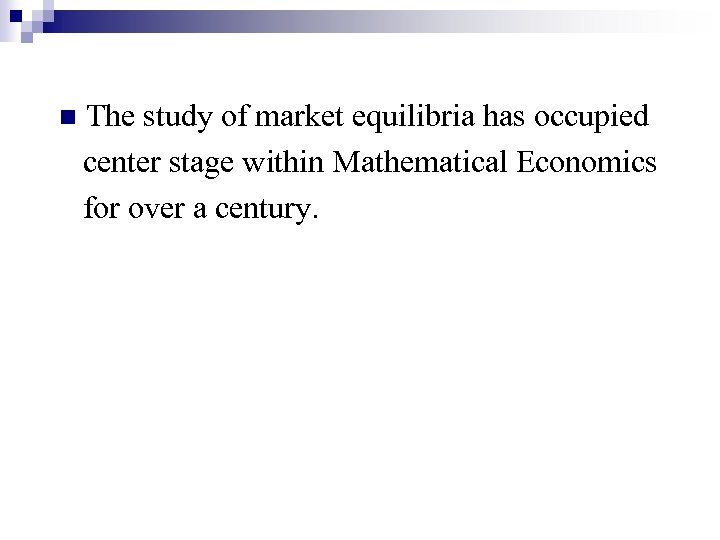n The study of market equilibria has occupied center stage within Mathematical Economics for over a century.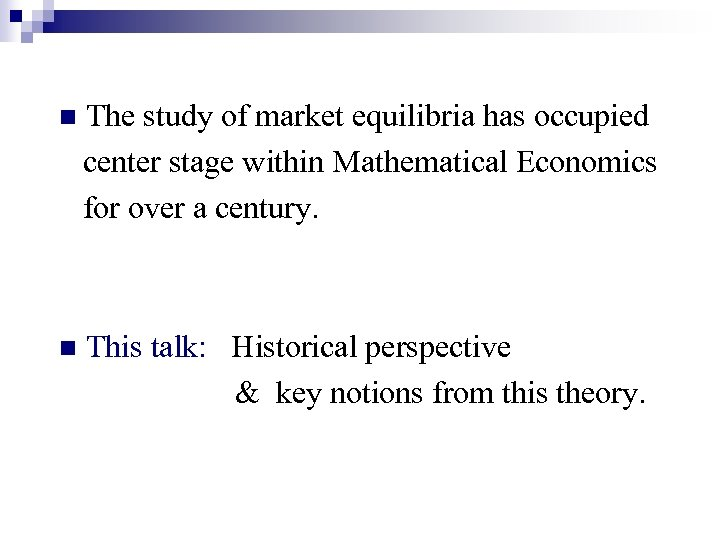n The study of market equilibria has occupied center stage within Mathematical Economics for over a century. n This talk: Historical perspective & key notions from this theory.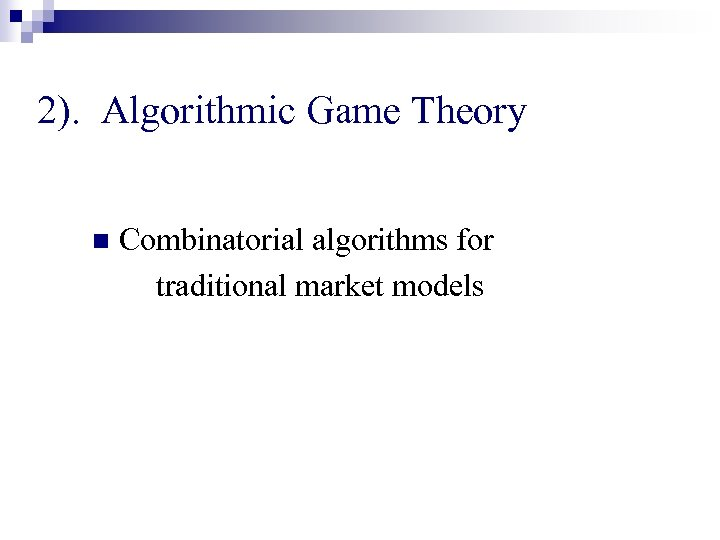2). Algorithmic Game Theory n Combinatorial algorithms for traditional market models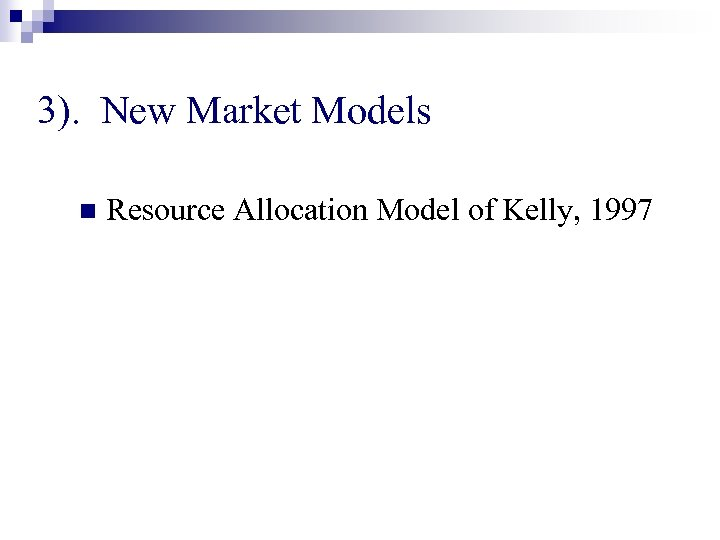3). New Market Models n Resource Allocation Model of Kelly, 1997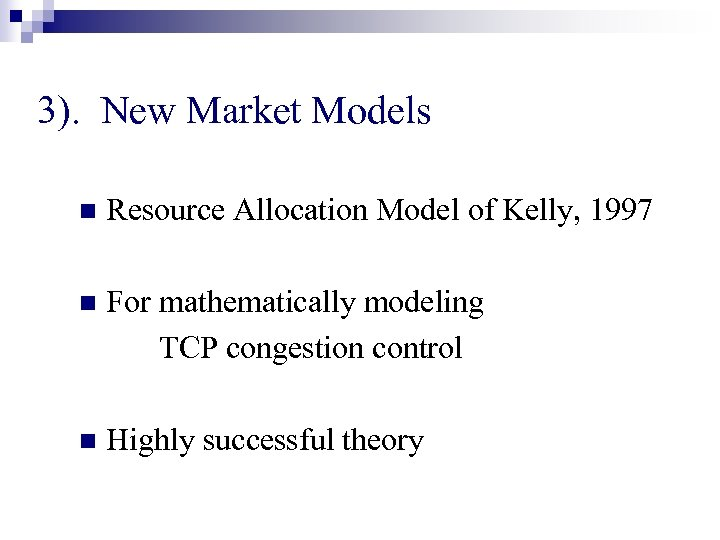3). New Market Models n Resource Allocation Model of Kelly, 1997 n For mathematically modeling TCP congestion control n Highly successful theory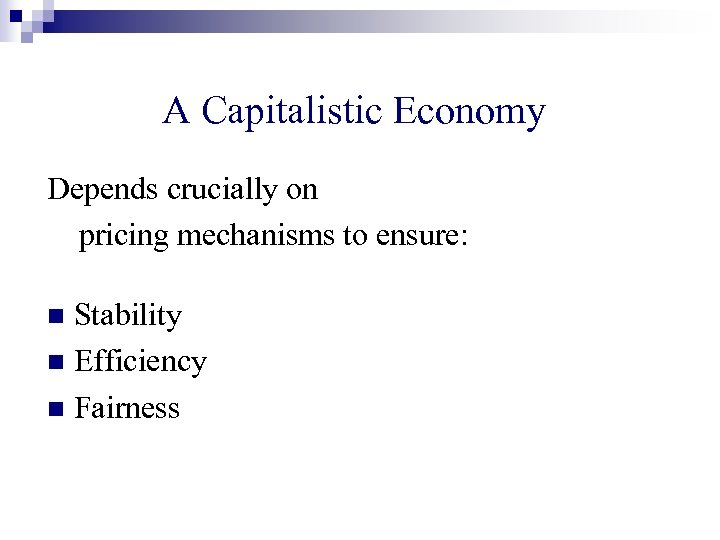A Capitalistic Economy Depends crucially on pricing mechanisms to ensure: Stability n Efficiency n Fairness n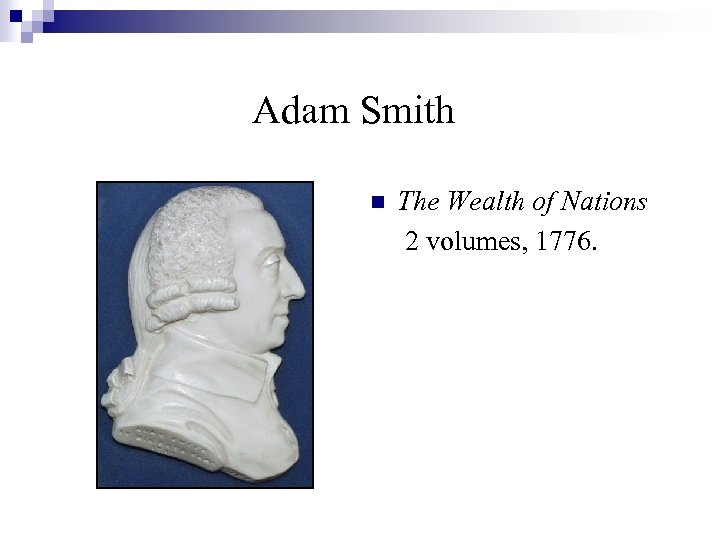Adam Smith n The Wealth of Nations 2 volumes, 1776.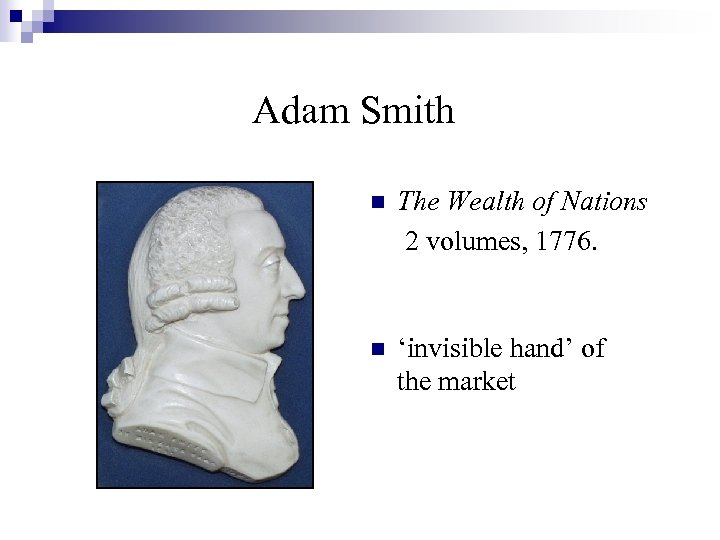Adam Smith n The Wealth of Nations 2 volumes, 1776. n ‘invisible hand’ of the market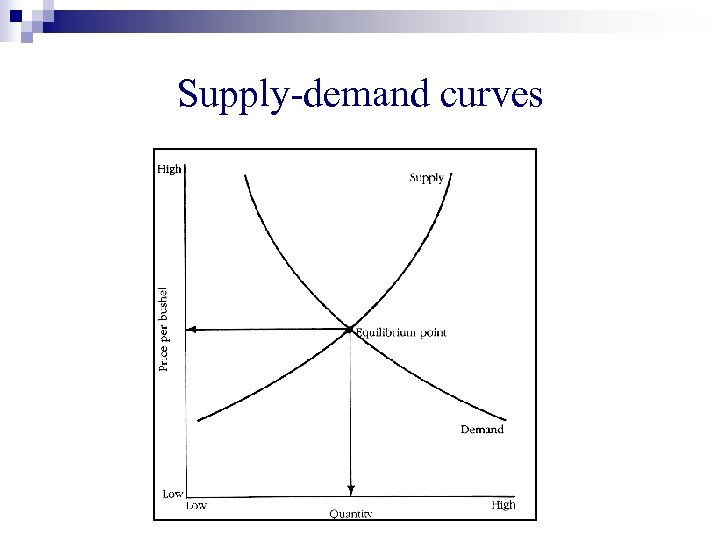Supply-demand curves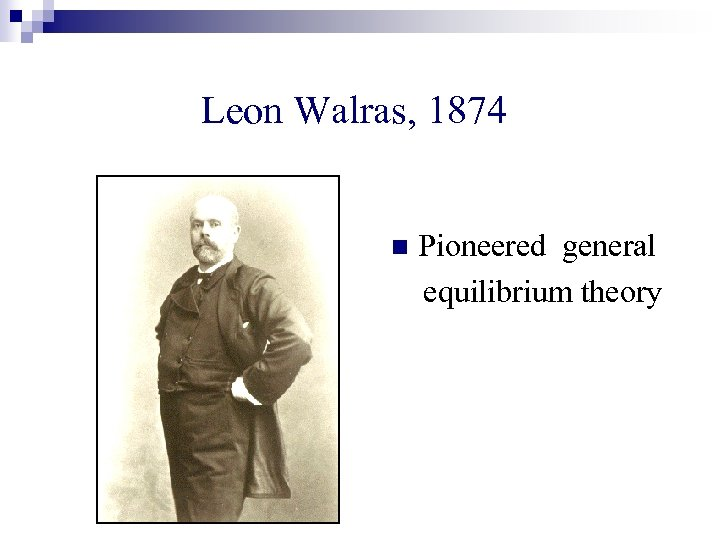Leon Walras, 1874 n Pioneered general equilibrium theory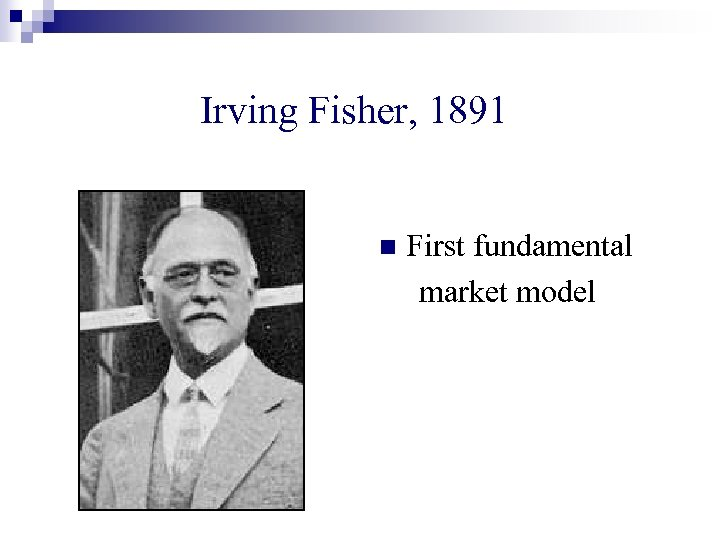Irving Fisher, 1891 n First fundamental market modelFisher’s Model, 1891 \$ \$\$\$\$\$ ¢ wine bread cheese n milk \$\$\$\$ People want to maximize happiness – assume Find prices linear utilities. s. t. market clears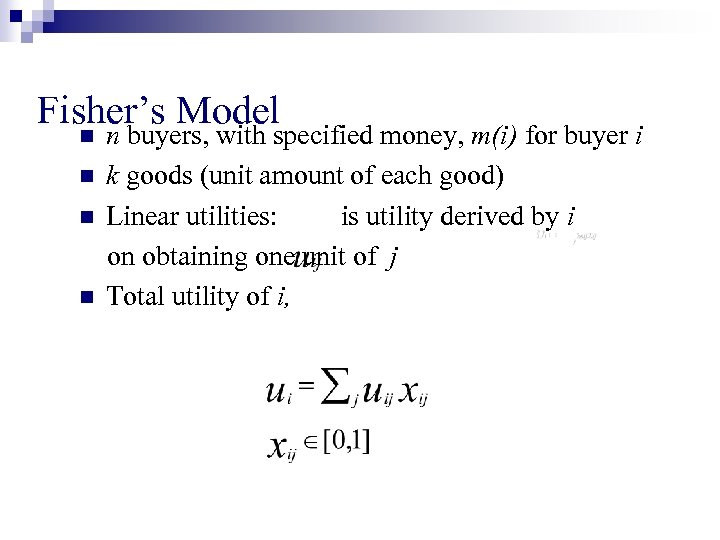Fisher’s Model n n n buyers, with specified money, m(i) for buyer i k goods (unit amount of each good) Linear utilities: is utility derived by i on obtaining one unit of j Total utility of i,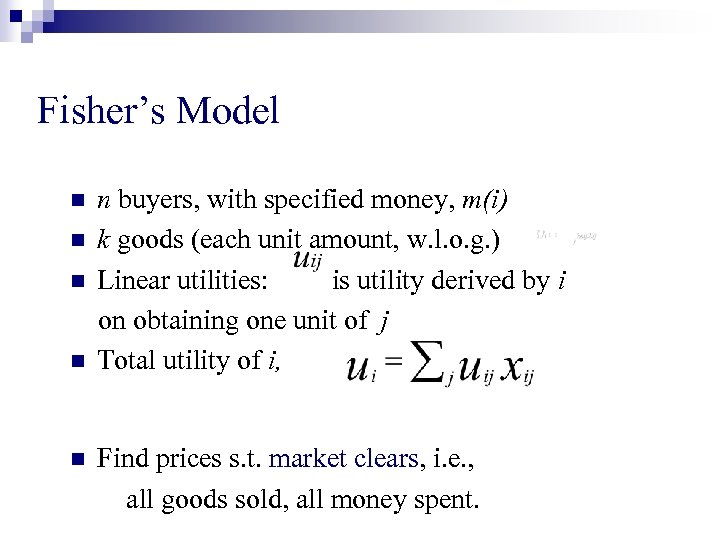Fisher’s Model n n n buyers, with specified money, m(i) k goods (each unit amount, w. l. o. g. ) Linear utilities: is utility derived by i on obtaining one unit of j Total utility of i, Find prices s. t. market clears, i. e. , all goods sold, all money spent.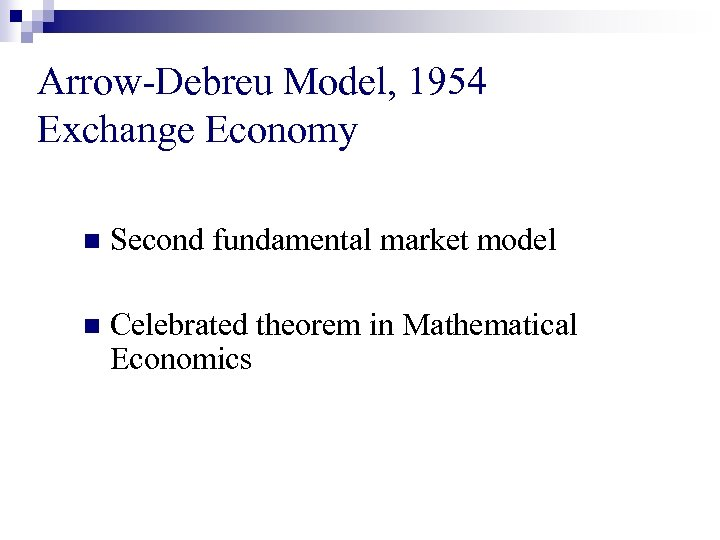Arrow-Debreu Model, 1954 Exchange Economy n Second fundamental market model n Celebrated theorem in Mathematical Economics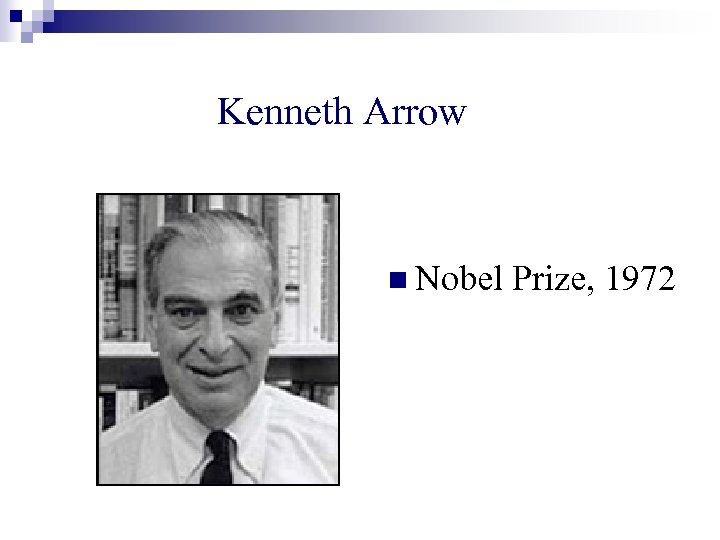Kenneth Arrow n Nobel Prize, 1972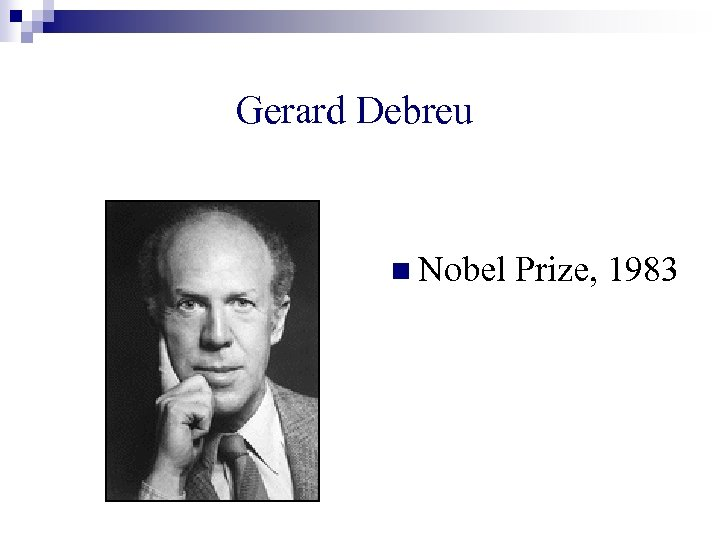Gerard Debreu n Nobel Prize, 1983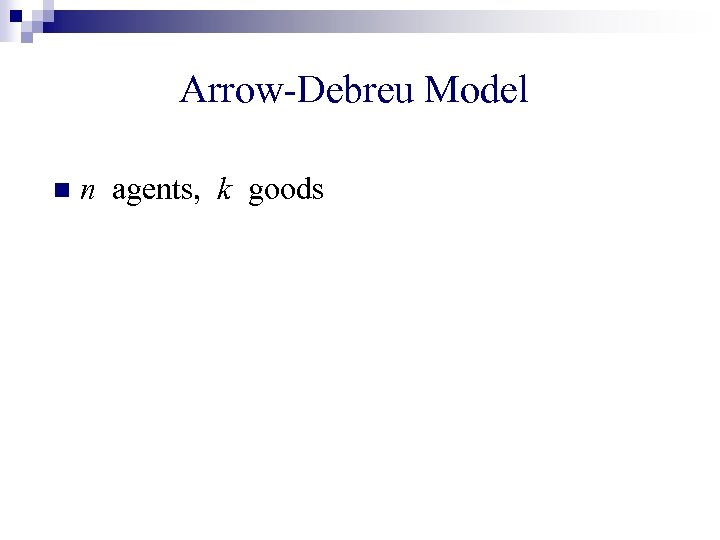Arrow-Debreu Model n n agents, k goods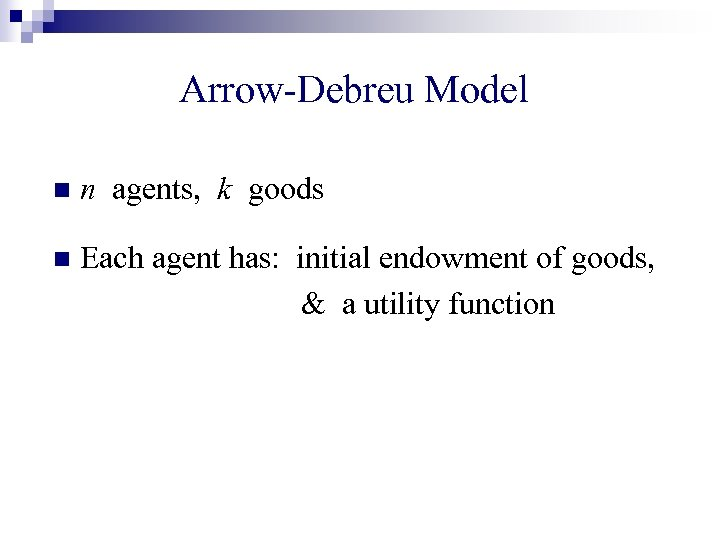Arrow-Debreu Model n n agents, k goods n Each agent has: initial endowment of goods, & a utility function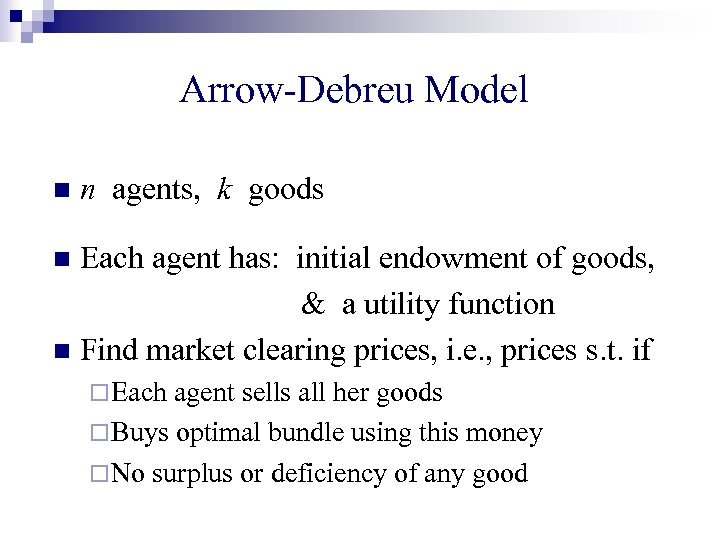Arrow-Debreu Model n n agents, k goods Each agent has: initial endowment of goods, & a utility function n Find market clearing prices, i. e. , prices s. t. if n ¨ Each agent sells all her goods ¨ Buys optimal bundle using this money ¨ No surplus or deficiency of any good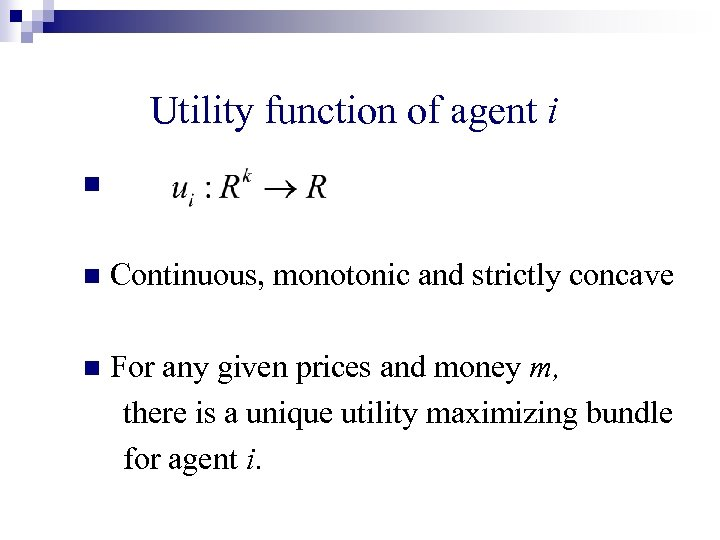Utility function of agent i n n Continuous, monotonic and strictly concave n For any given prices and money m, there is a unique utility maximizing bundle for agent i.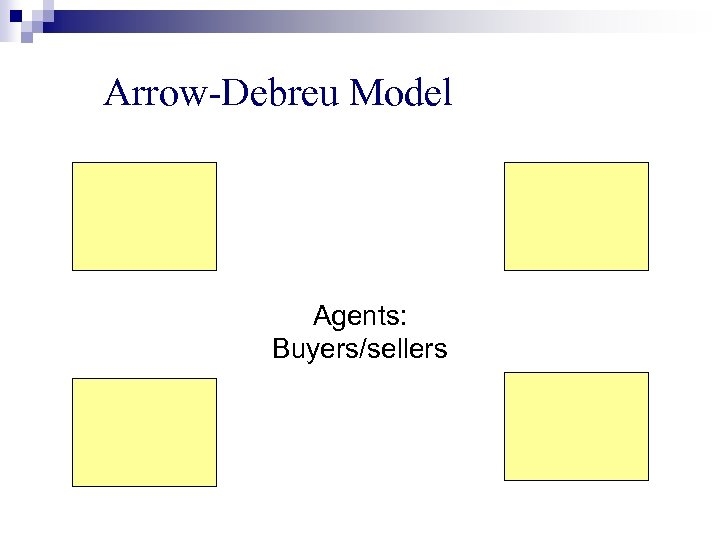Arrow-Debreu Model Agents: Buyers/sellers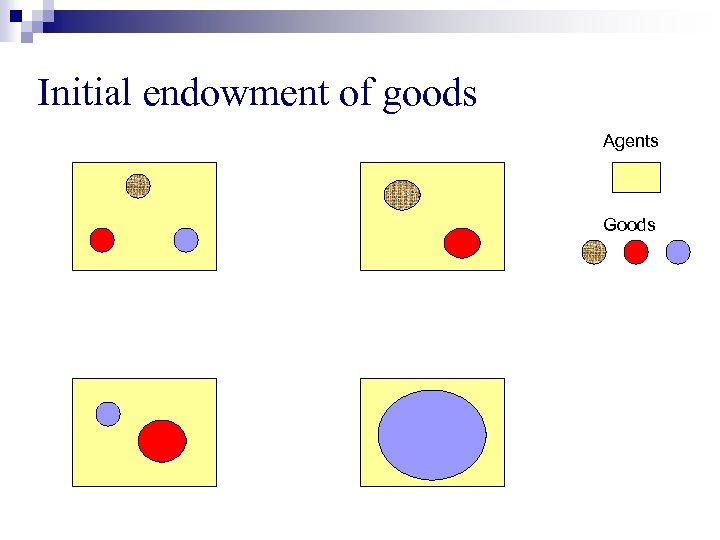Initial endowment of goods Agents Goods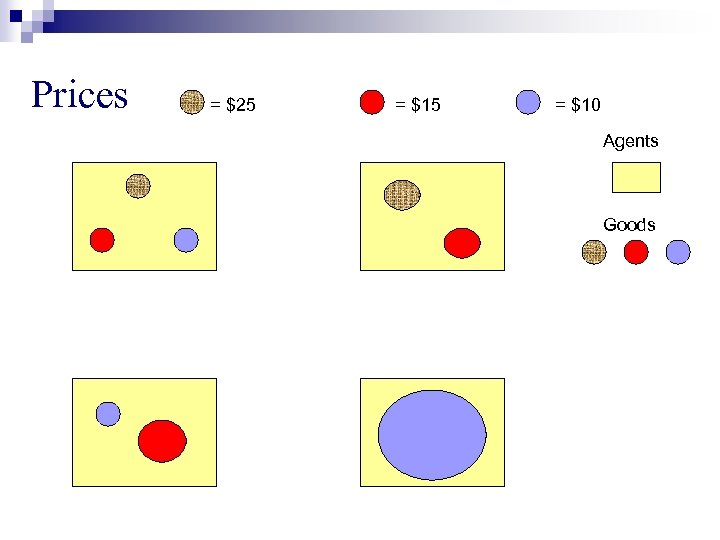Prices = \$25 = \$10 Agents Goods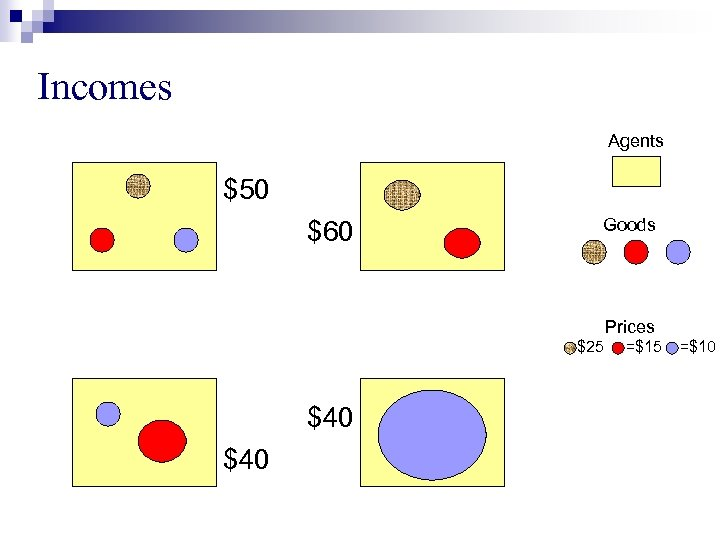Incomes Agents \$50 \$60 Goods Prices =\$25 \$40 =\$15 =\$10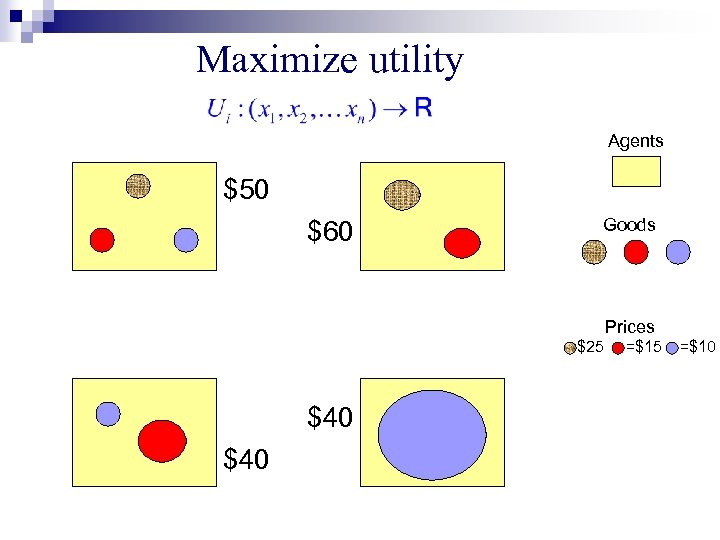Maximize utility Agents \$50 \$60 Goods Prices =\$25 \$40 =\$15 =\$10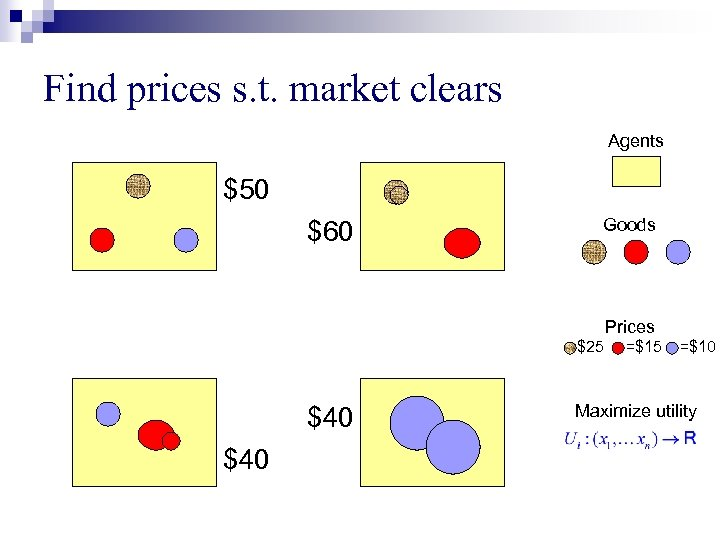Find prices s. t. market clears Agents \$50 \$60 Goods Prices =\$25 \$40 =\$15 =\$10 Maximize utility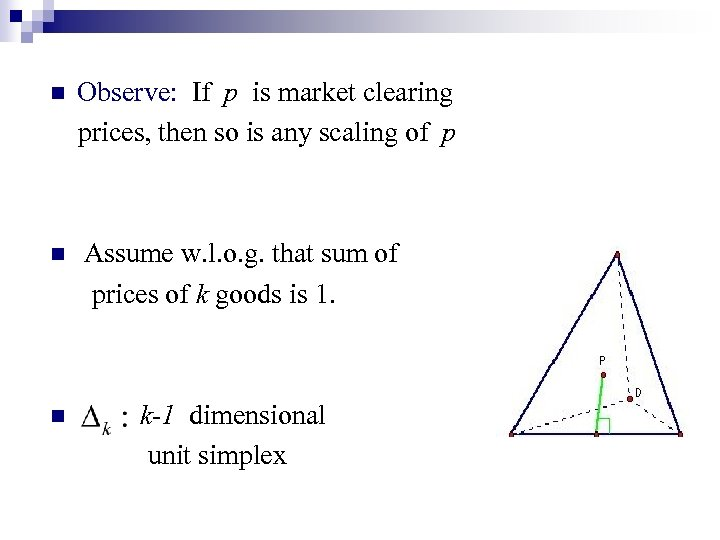n n n Observe: If p is market clearing prices, then so is any scaling of p Assume w. l. o. g. that sum of prices of k goods is 1. k-1 dimensional unit simplex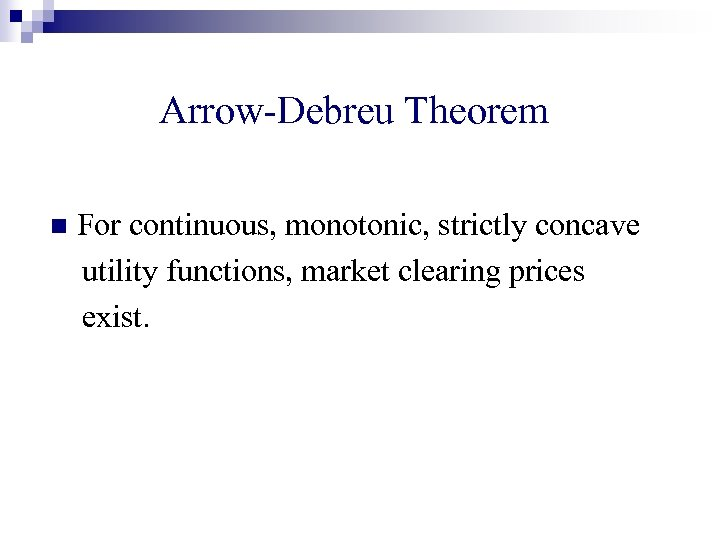Arrow-Debreu Theorem n For continuous, monotonic, strictly concave utility functions, market clearing prices exist.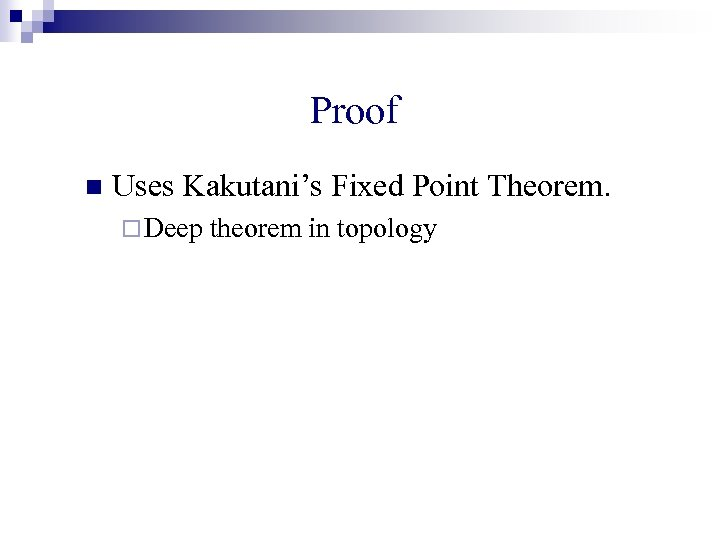Proof n Uses Kakutani’s Fixed Point Theorem. ¨ Deep theorem in topology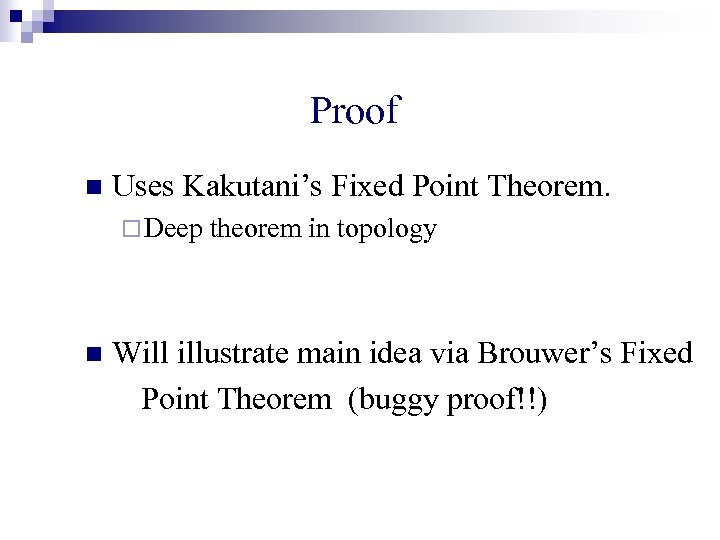Proof n Uses Kakutani’s Fixed Point Theorem. ¨ Deep n theorem in topology Will illustrate main idea via Brouwer’s Fixed Point Theorem (buggy proof!!)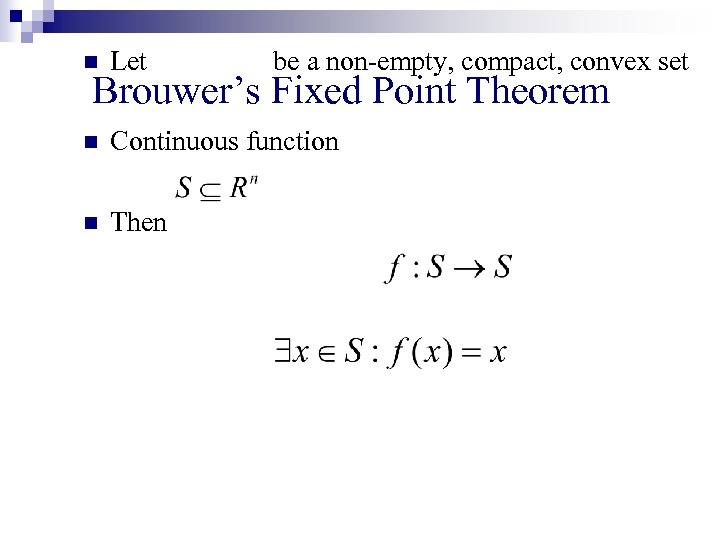n Let be a non-empty, compact, convex set n Continuous function n Then Brouwer’s Fixed Point Theorem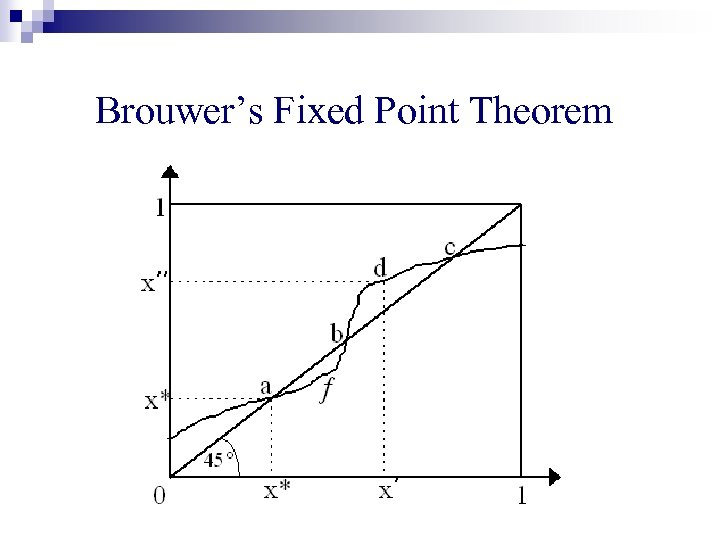Brouwer’s Fixed Point Theorem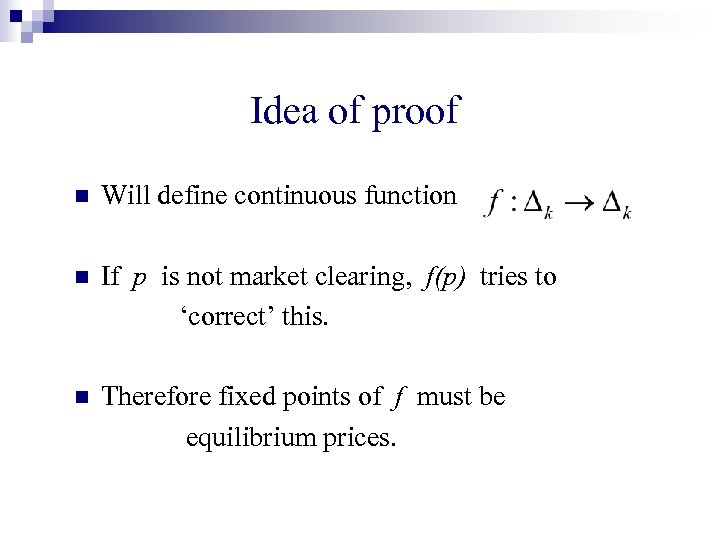Idea of proof n Will define continuous function n If p is not market clearing, f(p) tries to ‘correct’ this. n Therefore fixed points of f must be equilibrium prices.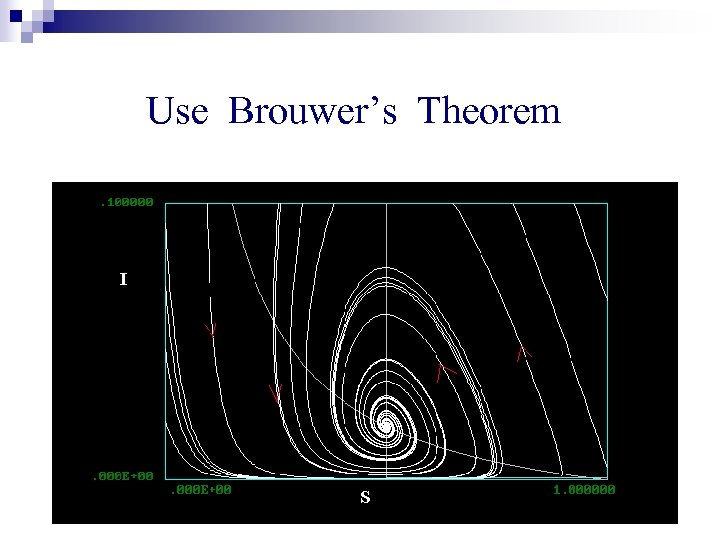Use Brouwer’s Theorem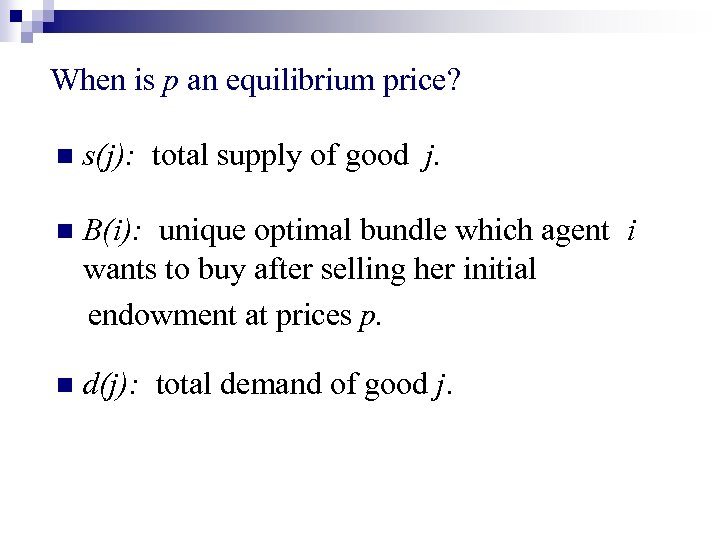When is p an equilibrium price? n s(j): total supply of good j. n B(i): unique optimal bundle which agent i wants to buy after selling her initial endowment at prices p. n d(j): total demand of good j.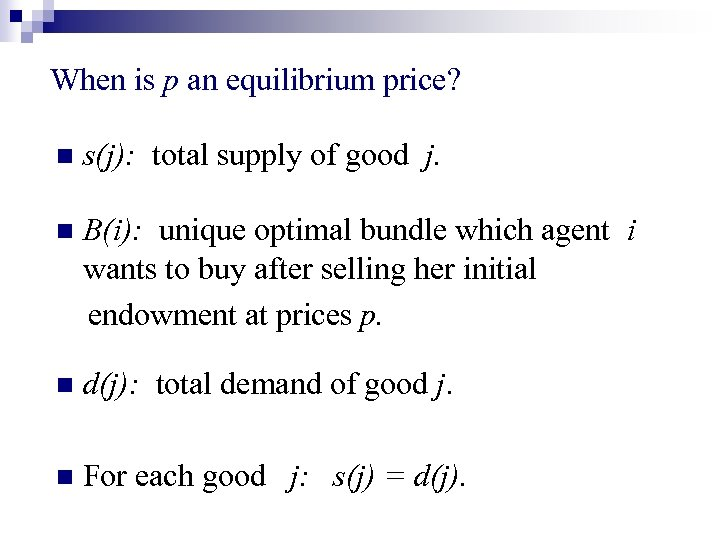When is p an equilibrium price? n s(j): total supply of good j. n B(i): unique optimal bundle which agent i wants to buy after selling her initial endowment at prices p. n d(j): total demand of good j. n For each good j: s(j) = d(j).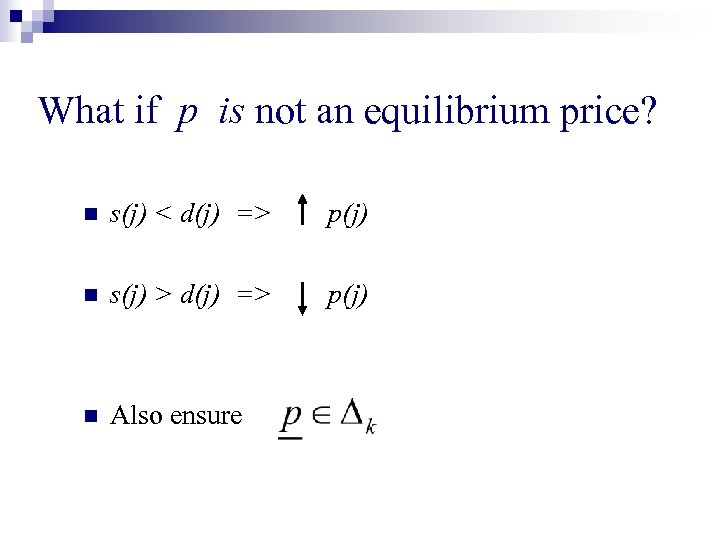What if p is not an equilibrium price? n s(j) < d(j) => p(j) n s(j) > d(j) => p(j) n Also ensure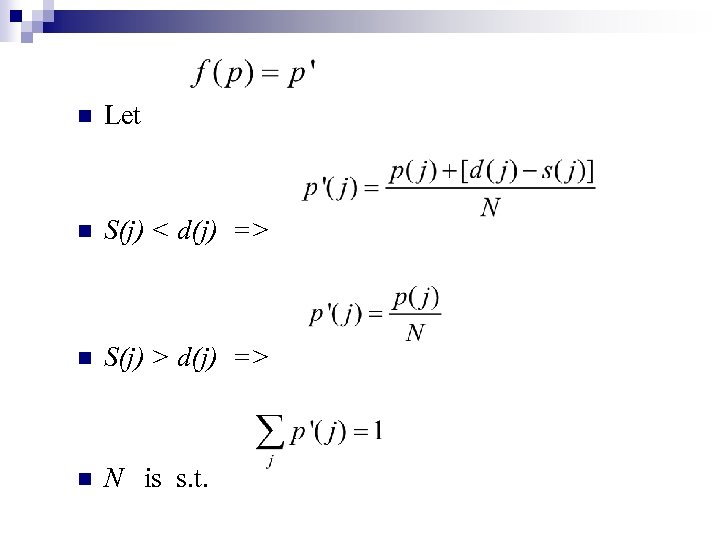n Let n S(j) < d(j) => n S(j) > d(j) => n N is s. t.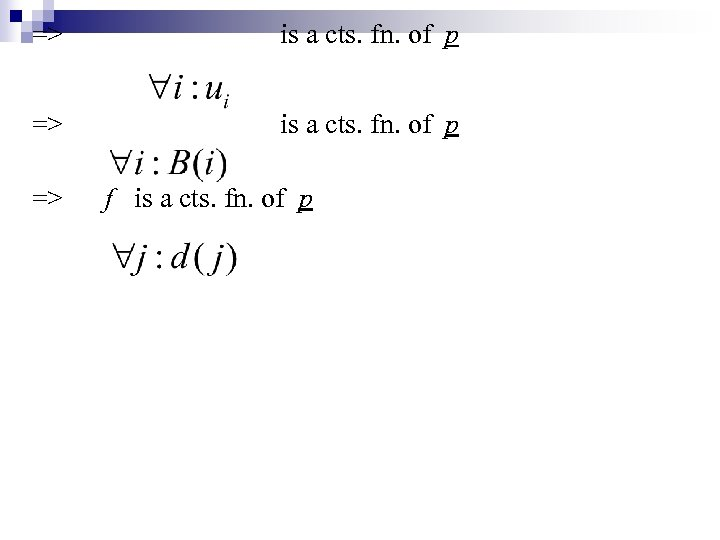=> is a cts. fn. of p => f is a cts. fn. of p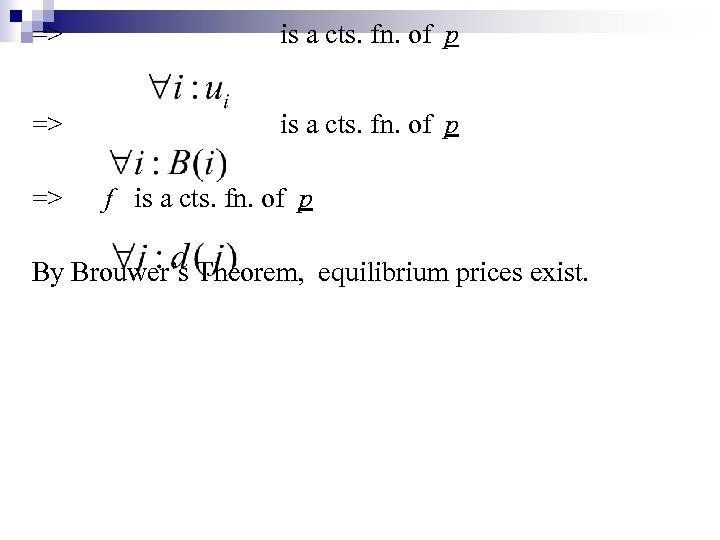=> is a cts. fn. of p => f is a cts. fn. of p By Brouwer’s Theorem, equilibrium prices exist.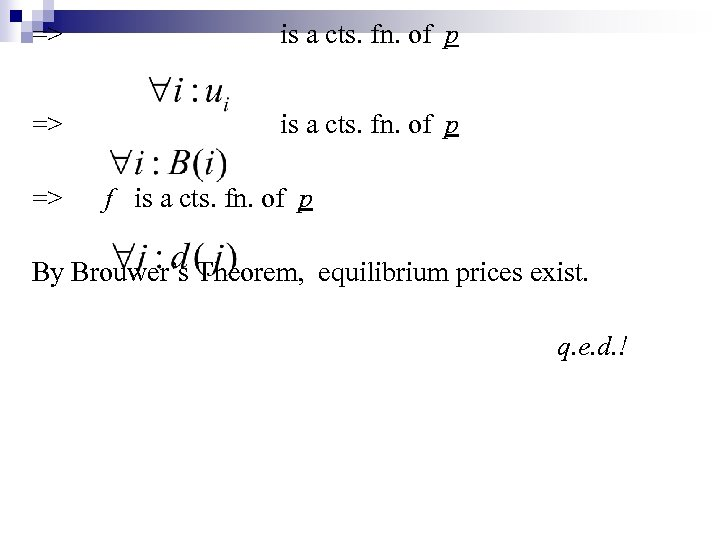=> is a cts. fn. of p => f is a cts. fn. of p By Brouwer’s Theorem, equilibrium prices exist. q. e. d. !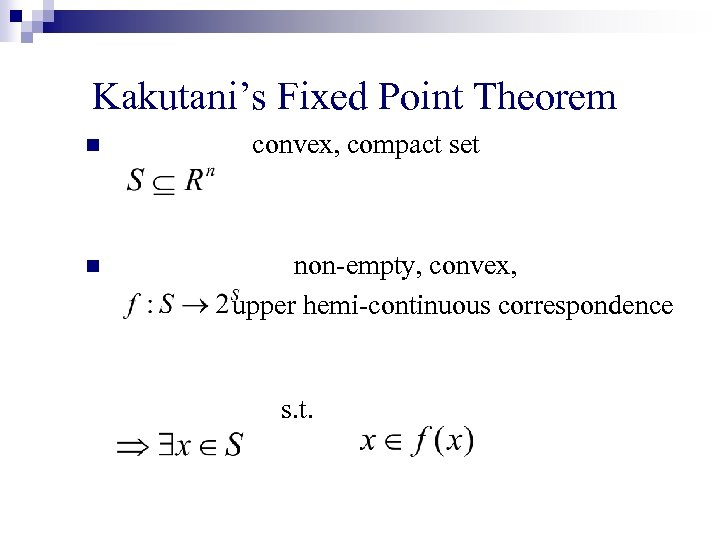Kakutani’s Fixed Point Theorem n n convex, compact set non-empty, convex, upper hemi-continuous correspondence s. t.Fisher reduces to Arrow-Debreu n Fisher: n buyers, k goods n AD: ¨ first n+1 agents n have money, utility for goods ¨ last agent has all goods, utility for money only.